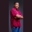Related Tags

scala
even numbers
array
communitycreator

# How to find even numbers in an array in ScalaTheodore Kelechukwu Onyejiaku

We use a while loop to find even numbers present in an array. All we need to do is use the modulus operator %. While we loop the array we will check if any element is divisible by two without a remainder. This is achieved by using the operator.

### Syntax

In Scala, the syntax for the while loop is given below:

while(condition){
// code body
}
Syntax for while loop

### Parameters

condition: This is a conditional statement. If it returns true, the code body will execute it. Otherwise, it won't.

### Code

object Main extends App {
// create default variables
var OurArray = Array(1, 5, 4, 2, 30, 22)
var counter = 0

// create a while loop
while(counter < OurArray.size) // if less than size of array
{
if(OurArray(counter) % 2 == 0) // if element is divisible by 2 and without remainder
{
// print element as even
println(OurArray(counter))
}

// increase counter after every loop
counter = counter + 1
}
}
Print out Even Numbers present in an Array in Scala

### Explanation

• Line 3: We create our array.
• Line 4: We create a counter. The counter will be used in our loop to keep a count of every loop.
• Line 7: We create a while loop. The counter should be less than the array size and the code body should run.
• Line 9: We check for every element if it is divisible by 2 using the modulus %. If the element is divisible by 2 and returns no remainder, then the element is printed. The element would be an even number.
• Line 16: We increase the counter for every loop so we can access each element of the array with OurArray(counter).

RELATED TAGS

scala
even numbers
array
communitycreator

CONTRIBUTORTheodore Kelechukwu Onyejiaku
RELATED COURSES

View all Courses

Keep Exploring

Learn in-demand tech skills in half the time